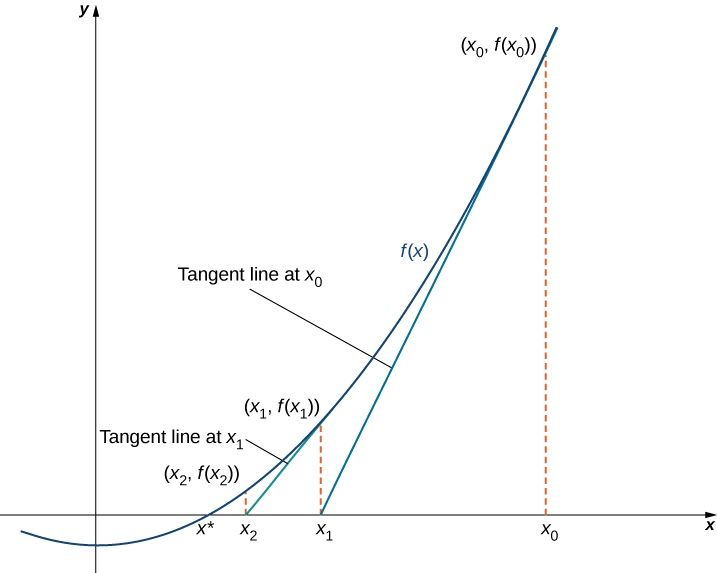# 4.9 Newton’s method

 Page 1 / 7
• Describe the steps of Newton’s method.
• Explain what an iterative process means.
• Recognize when Newton’s method does not work.
• Apply iterative processes to various situations.

In many areas of pure and applied mathematics, we are interested in finding solutions to an equation of the form $f\left(x\right)=0.$ For most functions, however, it is difficult—if not impossible—to calculate their zeroes explicitly. In this section, we take a look at a technique that provides a very efficient way of approximating the zeroes of functions . This technique makes use of tangent line approximations and is behind the method used often by calculators and computers to find zeroes.

## Describing newton’s method

Consider the task of finding the solutions of $f\left(x\right)=0.$ If $f$ is the first-degree polynomial $f\left(x\right)=ax+b,$ then the solution of $f\left(x\right)=0$ is given by the formula $x=-\frac{b}{a}.$ If $f$ is the second-degree polynomial $f\left(x\right)=a{x}^{2}+bx+c,$ the solutions of $f\left(x\right)=0$ can be found by using the quadratic formula. However, for polynomials of degree $3$ or more, finding roots of $f$ becomes more complicated. Although formulas exist for third- and fourth-degree polynomials, they are quite complicated. Also, if $f$ is a polynomial of degree $5$ or greater, it is known that no such formulas exist. For example, consider the function

$f\left(x\right)={x}^{5}+8{x}^{4}+4{x}^{3}-2x-7.$

No formula exists that allows us to find the solutions of $f\left(x\right)=0.$ Similar difficulties exist for nonpolynomial functions. For example, consider the task of finding solutions of $\text{tan}\left(x\right)-x=0.$ No simple formula exists for the solutions of this equation. In cases such as these, we can use Newton’s method to approximate the roots.

Newton’s method    makes use of the following idea to approximate the solutions of $f\left(x\right)=0.$ By sketching a graph of $f,$ we can estimate a root of $f\left(x\right)=0.$ Let’s call this estimate ${x}_{0}.$ We then draw the tangent line to $f$ at ${x}_{0}.$ If ${f}^{\prime }\left({x}_{0}\right)\ne 0,$ this tangent line intersects the $x$ -axis at some point $\left({x}_{1},0\right).$ Now let ${x}_{1}$ be the next approximation to the actual root. Typically, ${x}_{1}$ is closer than ${x}_{0}$ to an actual root. Next we draw the tangent line to $f$ at ${x}_{1}.$ If ${f}^{\prime }\left({x}_{1}\right)\ne 0,$ this tangent line also intersects the $x$ -axis, producing another approximation, ${x}_{2}.$ We continue in this way, deriving a list of approximations: ${x}_{0},{x}_{1},{x}_{2}\text{,…}.$ Typically, the numbers ${x}_{0},{x}_{1},{x}_{2}\text{,…}$ quickly approach an actual root $x*,$ as shown in the following figure.The approximations x 0 , x 1 , x 2 ,… approach the actual root x * . The approximations are derived by looking at tangent lines to the graph of f .

Now let’s look at how to calculate the approximations ${x}_{0},{x}_{1},{x}_{2}\text{,…}.$ If ${x}_{0}$ is our first approximation, the approximation ${x}_{1}$ is defined by letting $\left({x}_{1},0\right)$ be the $x$ -intercept of the tangent line to $f$ at ${x}_{0}.$ The equation of this tangent line is given by

$y=f\left({x}_{0}\right)+{f}^{\prime }\left({x}_{0}\right)\left(x-{x}_{0}\right).$

Therefore, ${x}_{1}$ must satisfy

$f\left({x}_{0}\right)+{f}^{\prime }\left({x}_{0}\right)\left({x}_{1}-{x}_{0}\right)=0.$

Solving this equation for ${x}_{1},$ we conclude that

${x}_{1}={x}_{0}-\frac{f\left({x}_{0}\right)}{f\prime \left({x}_{0}\right)}.$

Similarly, the point $\left({x}_{2},0\right)$ is the $x$ -intercept of the tangent line to $f$ at ${x}_{1}.$ Therefore, ${x}_{2}$ satisfies the equation

${x}_{2}={x}_{1}-\frac{f\left({x}_{1}\right)}{f\prime \left({x}_{1}\right)}.$

In general, for $n>0,{x}_{n}$ satisfies

${x}_{n}={x}_{n-1}-\frac{f\left({x}_{n-1}\right)}{f\prime \left({x}_{n-1}\right)}.$

Next we see how to make use of this technique to approximate the root of the polynomial $f\left(x\right)={x}^{3}-3x+1.$

any genius online ? I need help!!
Pina
need to learn polynomial
Zakariya
i will teach...
nandu
I'm waiting
Zakariya
evaluate the following computation (x³-8/x-2)
teach me how to solve the first law of calculus.
what is differentiation
f(x) = x-2 g(x) = 3x + 5 fog(x)? f(x)/g(x)
fog(x)= f(g(x)) = x-2 = 3x+5-2 = 3x+3 f(x)/g(x)= x-2/3x+5
diron
pweding paturo nsa calculus?
jimmy
how to use fundamental theorem to solve exponential
find the bounded area of the parabola y^2=4x and y=16x
what is absolute value means?
Chicken nuggets
Hugh
🐔
MM
🐔🦃 nuggets
MM
(mathematics) For a complex number a+bi, the principal square root of the sum of the squares of its real and imaginary parts, √a2+b2 . Denoted by | |. The absolute value |x| of a real number x is √x2 , which is equal to x if x is non-negative, and −x if x is negative.
Ismael
find integration of loge x
find the volume of a solid about the y-axis, x=0, x=1, y=0, y=7+x^3
how does this work
Can calculus give the answers as same as other methods give in basic classes while solving the numericals?
log tan (x/4+x/2)
Rohan
Rohan
y=(x^2 + 3x).(eipix)
Claudia
Ismael
A Function F(X)=Sinx+cosx is odd or even?
neither
David
Neither
Lovuyiso
f(x)=1/1+x^2 |=[-3,1]
apa itu?
fauzi
determine the area of the region enclosed by x²+y=1,2x-y+4=0
Hi
MP
Hi too
Vic
hello please anyone with calculus PDF should share
Which kind of pdf do you want bro?
Aftab
hi
Abdul
can I get calculus in pdf
Abdul
explain for me
Usman
okay I have such documents
Fitzgerald
Hamza

#### Get Jobilize Job Search Mobile App in your pocket Now!By Robert MurphyBy Sarah WarrenBy Stephen VoronBy Vanessa SoledadBy Heather McAvoyBy Maureen MillerBy OpenStaxBy Yacoub JayoghliBy Cath YuBy Stephen Voron